# Swift 中 Enum 及 Optional 介绍# Enum

## 枚举的基本用法

`swift` 中通过 `enum` 关键字来声明一个枚举

``````enum CXEnum {
case test_one
case test_two
case test_three
}
``````

``````typedef NS_ENUM(NSUInteger, CXEnum) {
A,
B,
C,
};
``````

`Swift` 中的枚举则更加灵活，并且不需给枚举中的每一个成员都提供值。如果一个值(所谓“原 始”值)要被提供给每一个枚举成员，那么这个值可以是字符串、字符、任意的整数值，或者是浮点类型。

``````enum Color : String {
case red = "Red"
case amber = "Amber"
case green = "Green"
}

enum CXEnum: Double {
case a = 10.0
case b= 20.0
case c = 30.0
case d = 40.0
}
``````

``````enum DayOfWeek: Int {
case mon, tue, wed, thu, fri = 10, sat, sun
}
``````

``````enum DayOfWeek: String {
case mon, tue, wed, thu, fri = "Hello world", sat, sun
}
``````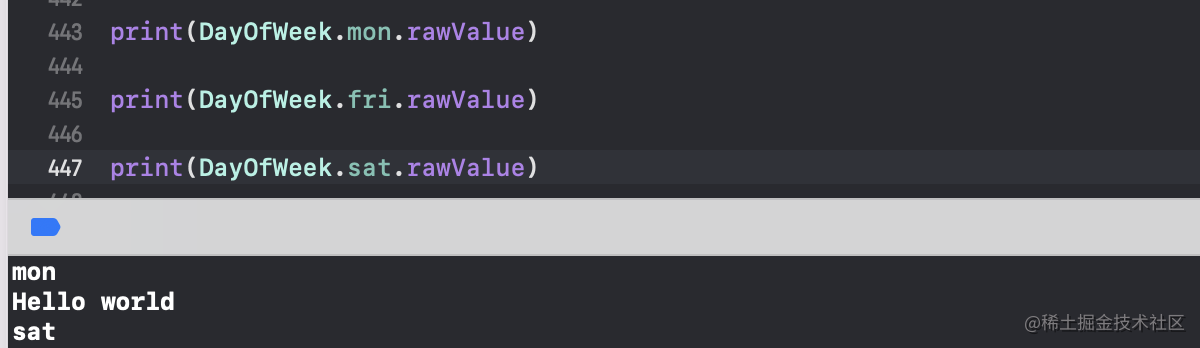## 关联值

``````enum Shape {
case rectangle(width: Int, height: Int)
}

var rectangle = Shape.rectangle(width: 15, height: 7)
``````

``````enum Weak: String {
case MONDAY
case TUEDAY
case WEDDAY
case THUDAY
case FRIDAY
case SATDAY
case SUNDAY
}

let currentWeak: Weak = Weak.MONDAY
switch currentWeak {
case .MONDAY: print(Weak.MONDAY.rawValue)
case .TUEDAY: print(Weak.TUEDAY.rawValue)
case .WEDDAY: print(Weak.WEDDAY.rawValue)
case .THUDAY: print(Weak.THUDAY.rawValue)
case .FRIDAY: print(Weak.FRIDAY.rawValue)
case .SUNDAY: print(Weak.SUNDAY.rawValue)
case .SATDAY: print(Weak.SUNDAY.rawValue)
}
``````

``````switch currentWeak{
case .SATDAY, .SUNDAY: print("Happy Day")
}
``````

``````enum Shape {
case rectangle(width: Int, height: Int)
}

switch shape {
case let .rectangle(width, height):
print("rectangle width:\(width),height\(height)")
}
``````

``````switch shape{
case .rectangle(let width, var height):
height += 10
print("rectangle width:\(width),height\(height)")
}
``````

## 枚举的大小

``````enum Weak: String {
case MONDAY
case TUEDAY
case WEDDAY
case THUDAY
case FRIDAY
case SATDAY
case SUNDAY
}
``````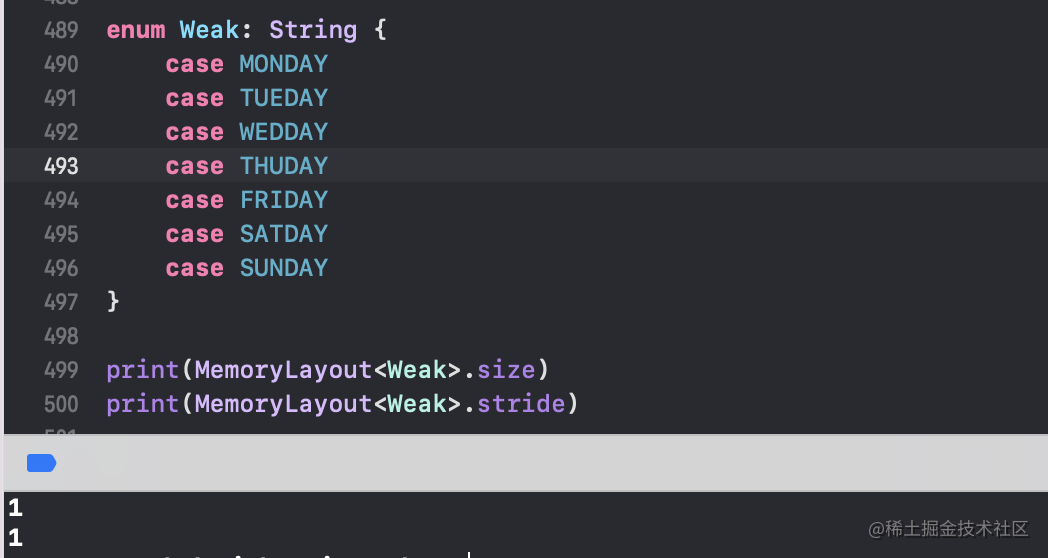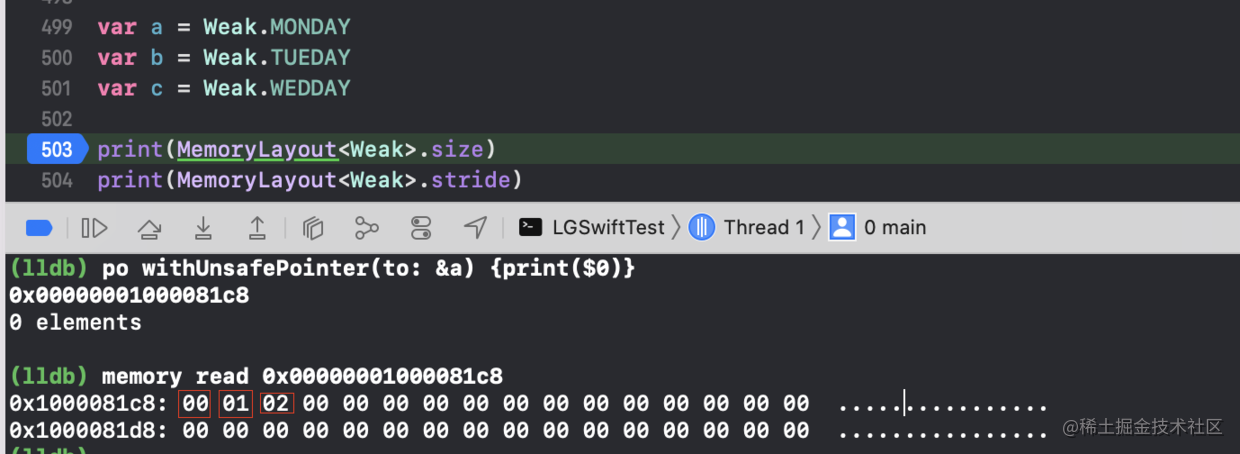`No-payload enums` 的布局比较简单，我们也比较好理解，接下来我们来理解一下 `Single-payload enums` 的内存布局， 字面意思就是有一个成员负载的 `enum`，比如下面这个例子：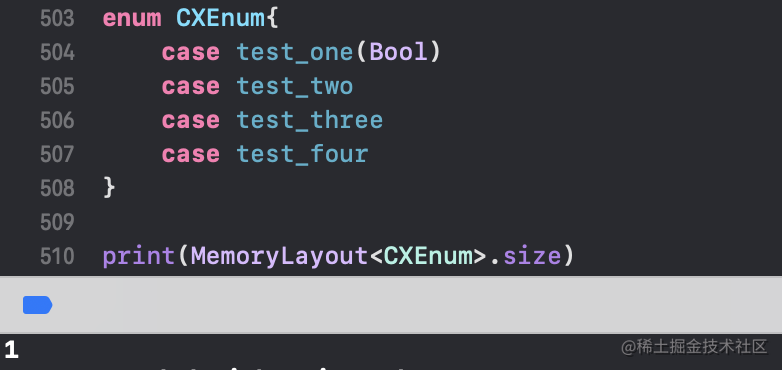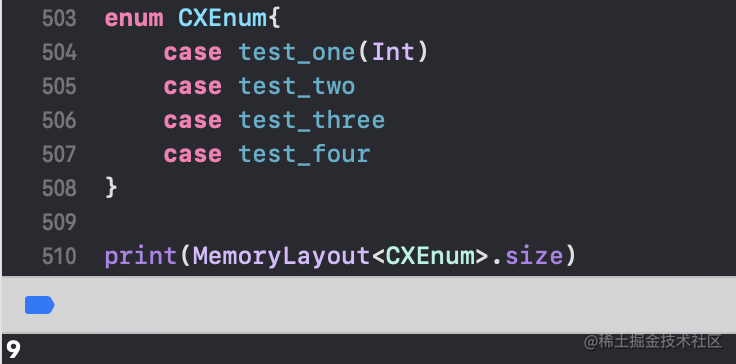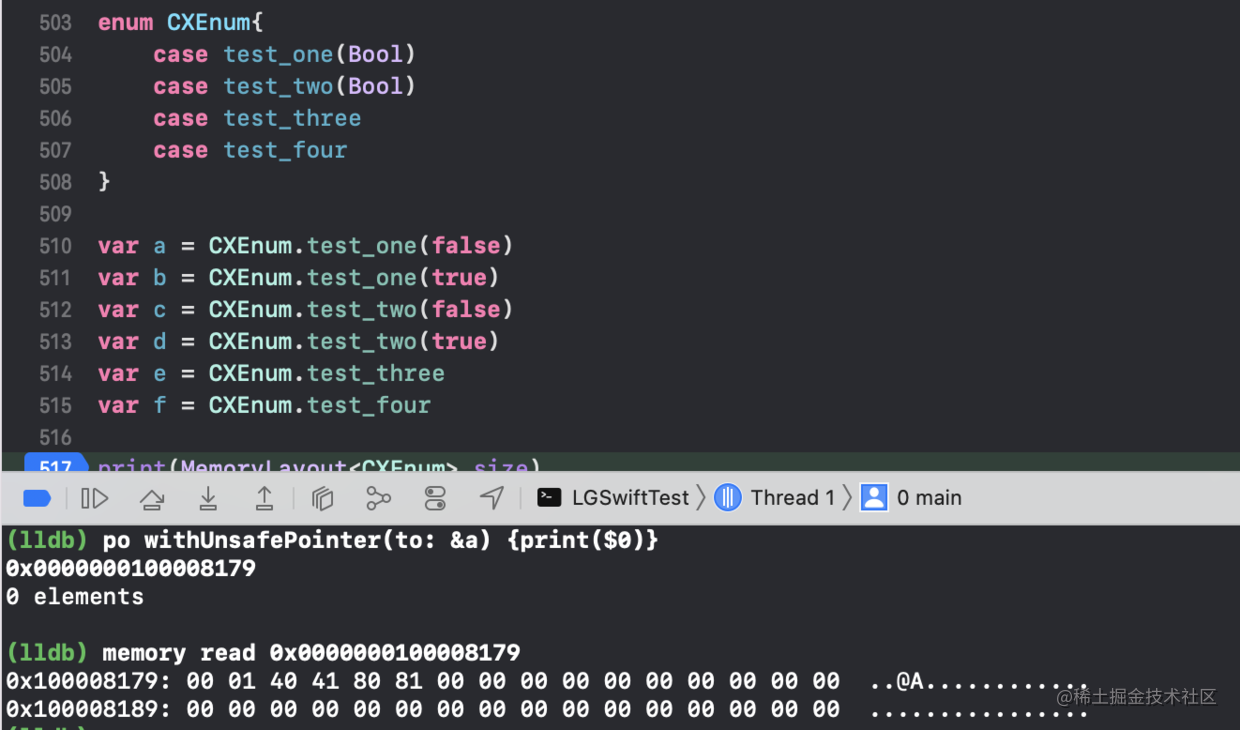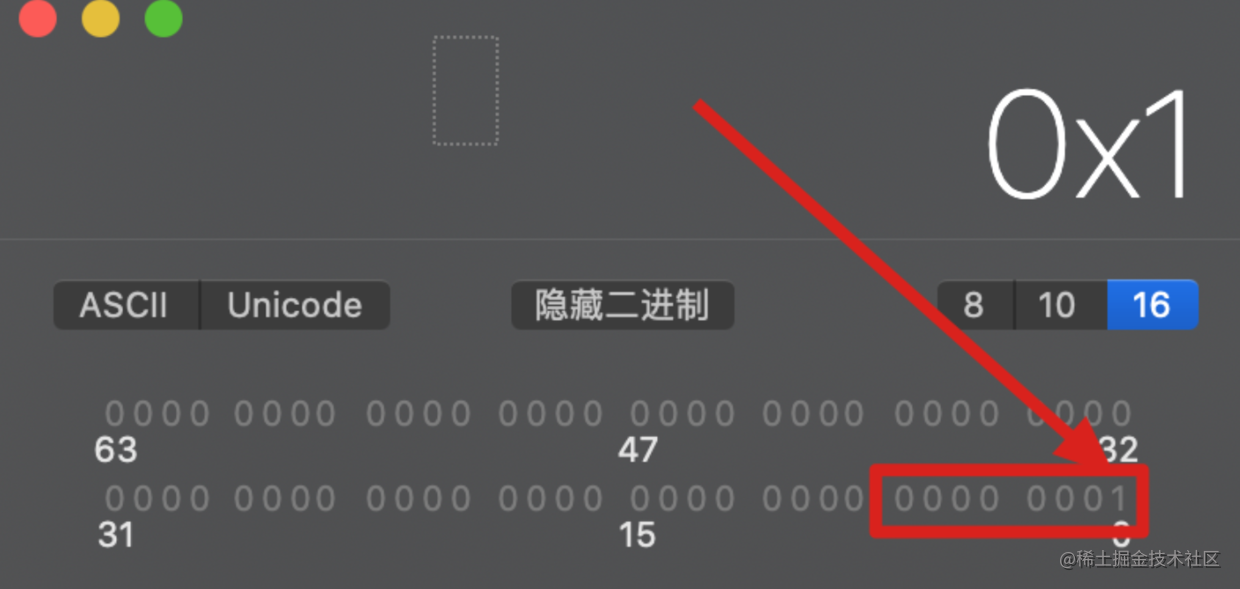``````enum CXEnum{
case test_one(Bool)
case test_two(Int)
case test_three
case test_four
}
``````

``````enum CXEnum{
case test_one(Bool)
case test_two(Int, Int, Int)
case test_three
case test_four
}
``````

``````enum CXEnum{
case test_one
}
``````

### indirect 关键字

``````indirect enum BinaryTree<T> {
case empty
case node(left: BinaryTree, value: T, right: BinaryTree)
}
``````

``````enum BinaryTree<T> {
case empty
indirect case node(left: BinaryTree, value: T, right: BinaryTree)
}
``````

# Optional

## 认识可选值

``````class LGTeacher{
var age: Int?
}
``````

``````var age: Int? = var age: Optional<Int>
``````

``````@frozen
public enum Optional<Wrapped>: ExpressibleByNilLiteral {
case none
case some(Wrapped)
}
``````

``````enum MyOptional<Value> {
case some(Value)
case none
}
``````

``````enum MyOptional<Value> {
case some(Value)
case none
}

func getOddValue(_ value: Int) -> MyOptional<Int> {
if value % 2 == 0 {
return .some(value) }
else{
return .none
}
}
``````

``````var array = [1, 2, 3, 4, 5, 6]
for element in array {
let value = getOddValue(element)
array.remove(at: array.firstIndex(of: value))
}
``````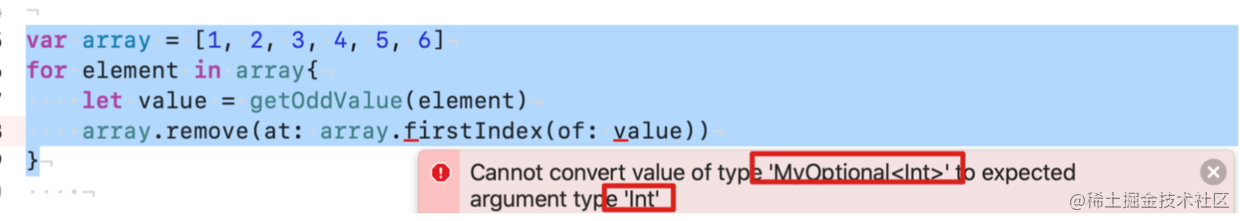``````for element in array {
let value = getOddValue(element)
switch value {
case .some(let value):
array.remove(at: array.firstIndex(of: value)!)
case .none:
print("vlaue not exist") }
}
``````

``````func getOddValue(_ value: Int) -> Int? {
if value % 2 == 0 {
return .some(value)
}else{
return .none
}
}
``````

``````if let value = value{
array.remove(at: array.firstIndex(of: value)!)
}
``````

`gurad let``if let` 刚好相反，守护一定有值。如果没有，直接返回。通常判断是否有值之后，会做具体的逻辑实现，通常代码多，如果用 `if let` 凭空多了一层分支，`guard let` 是降低分支层次的办法。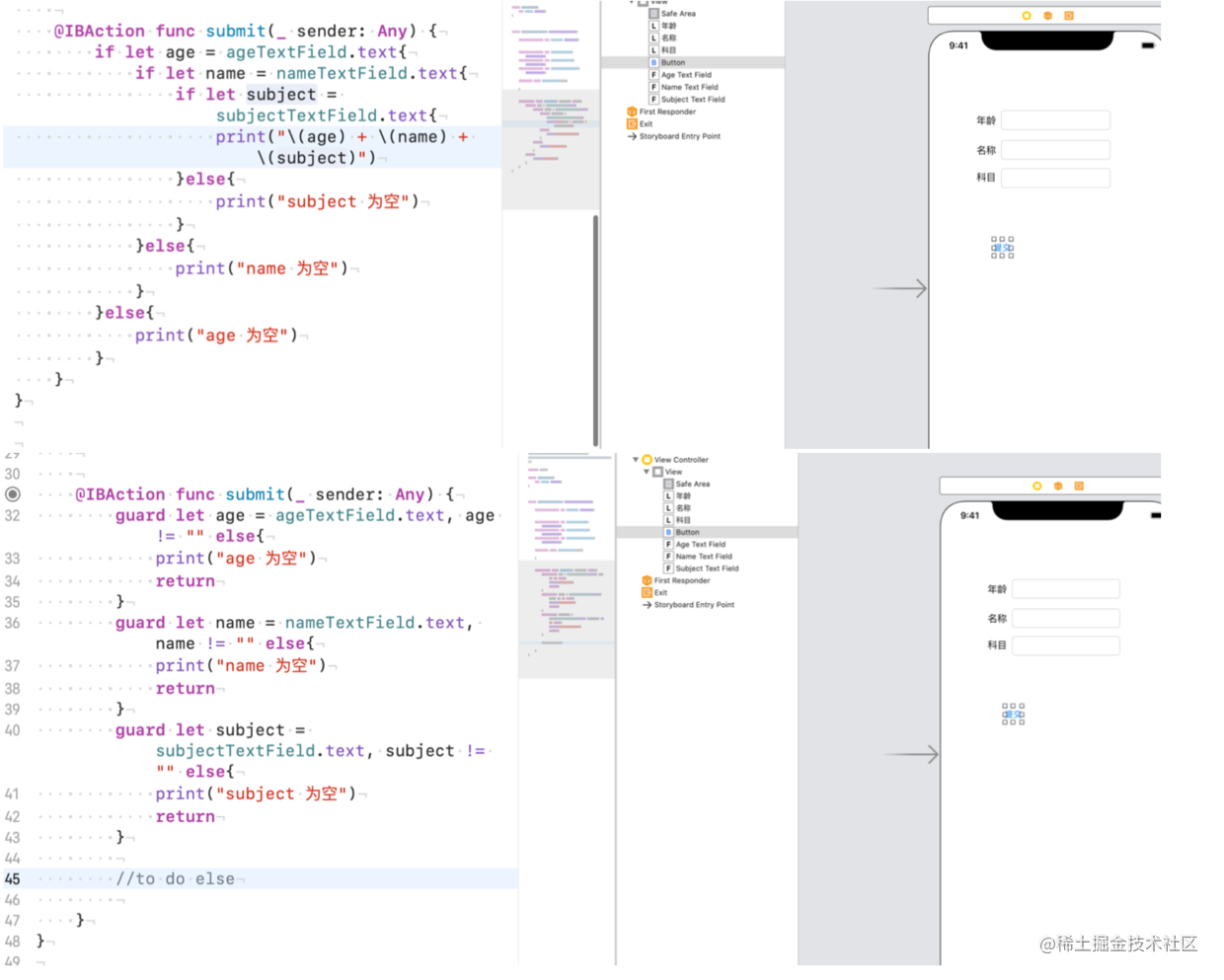## 可选链

``````let str: String? = "abc"
let upperStr = str?.uppercased() // Optional<"ABC">
var str: String?
let upperStr = str?.uppercased() // nil
``````

``````let str: String? = "kody"
let upperStr = str?.uppercased().lowercased()
``````

``````var closure: ((Int) -> ())? closure?(1) // closure 为 nil 不执行
let dict = ["one": 1, "two": 2] dict?["one"] // Optional(1) dict?["three"] // nil
``````

## ?? 运算符 (空合并运算符)

`( a ?? b )` 将对可选类型 `a` 进行空判断，如果 `a` 包含一个值就进行解包，否则就返回 一个默认值 `b`

• 表达式 `a` 必须是 `Optional` 类型
• 默认值 `b` 的类型必须要和 `a` 存储值的类型保持一致

## 运算符重载

``````struct Vector {
let x: Int
let y: Int
}

extension Vector {
static func + (fistVector: Vector, secondVector: Vector) -> Vector {
return Vector(x: fistVector.x + secondVector.x, y: fistVector.y + seco
}
static prefix func - (vector: Vector) -> Vector {
return Vector(x: -vector.x, y: -vector.y)
}
static func - (fistVector: Vector, secondVector: Vector) -> Vector {
return fistVector + -secondVector
}
}
``````

## 隐士解析可选类型

``````var age: Int?
var age1: Int!
age = nil
age1 = nil
``````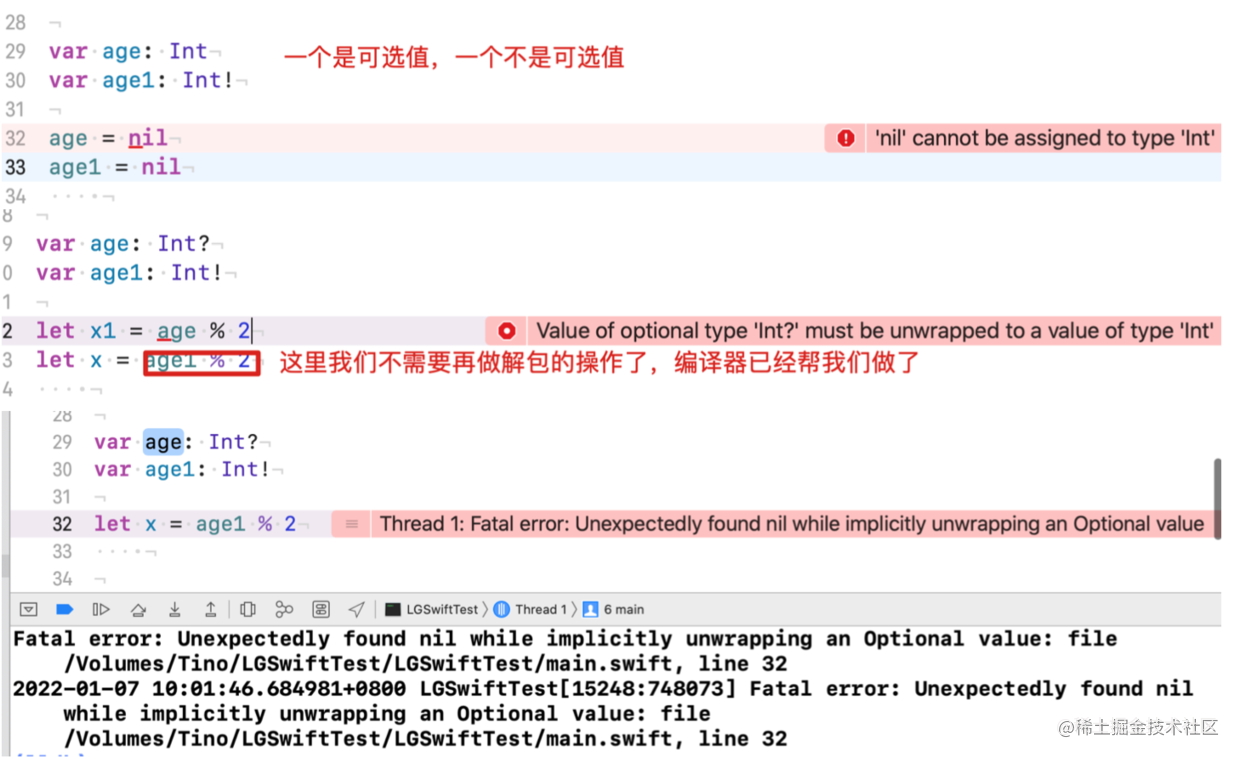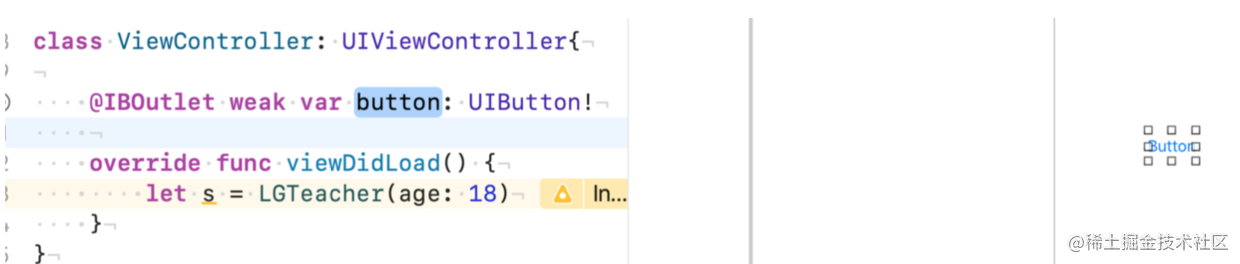`IBOutlet` 类型是 `Xcode` 强制为可选类型的，因为它不是在初始化时赋值的，而是在加载视图的时候。你可以把它设置为普通可选类型，但是如果这个视图加载正确，它是不会为空的。

## 与可选值有关的高阶函数

• `map` : 这个方法接受一个闭包，如果可选值有内容则调用这个闭包进行转换
``````var dict = ["one": "1", "two": "2"]
let result = dict["one"].map{ Int(\$0) } // Optional(Optional(1))
``````

• `flatMap`: 可以把结果展平成为单个可选值
``````var dict = ["one": "1", "two": "2"]
let result = dict["one"].flatMap{ Int(\$0) } // Optional(1)
``````
• 注意，这个方法是作用在 `Optioanl` 上的

• 作用在 `Sequence` 上的 `flatMap` 方法在 `Swift4.1` 中被更名为 `compactMap`，该方法可以将序列中的 `nil` 过滤出去。

``````let array = ["1", "2", "3", nil]
let result = array.compactMap{ \$0 } // ["1", "2", "3"]
let array = ["1", "2", "3", "four"]
let result = array.compactMap{ Int(\$0) } // [1, 2, 3]
``````

iOS

iOS